# Frequency and time-frequency sensor analysis#

The objective is to show you how to explore the spectral content of your data (frequency and time-frequency). Here we’ll work on Epochs.

We will use this dataset: Somatosensory. It contains so-called event related synchronizations (ERS) / desynchronizations (ERD) in the beta band.

```# Authors: Alexandre Gramfort <alexandre.gramfort@inria.fr>
#          Stefan Appelhoff <stefan.appelhoff@mailbox.org>
#          Richard Höchenberger <richard.hoechenberger@gmail.com>
#
```
```import matplotlib.pyplot as plt
import numpy as np

import mne
from mne.datasets import somato
from mne.time_frequency import tfr_morlet
```

Set parameters

```data_path = somato.data_path()
subject = "01"

# Setup for reading the raw data
# crop and resample just to reduce computation time
events = mne.find_events(raw, stim_channel="STI 014")

picks = mne.pick_types(raw.info, meg="grad", eeg=False, eog=True, stim=False)

# Construct Epochs
event_id, tmin, tmax = 1, -1.0, 3.0
baseline = (None, 0)
epochs = mne.Epochs(
raw,
events,
event_id,
tmin,
tmax,
picks=picks,
baseline=baseline,
)
```
```Opening raw data file /home/circleci/mne_data/MNE-somato-data/sub-01/meg/sub-01_task-somato_meg.fif...
Range : 237600 ... 506999 =    791.189 ...  1688.266 secs
Reading 0 ... 72074  =      0.000 ...   240.001 secs...
29 events found on stim channel STI 014
Event IDs: 
29 events found on stim channel STI 014
Event IDs: 
29 events found on stim channel STI 014
Event IDs: 
29 matching events found
Setting baseline interval to [-1.0, 0.0] s
Applying baseline correction (mode: mean)
0 projection items activated
Using data from preloaded Raw for 29 events and 801 original time points ...
Rejecting  epoch based on EOG : ['EOG 061']
```

## Frequency analysis#

We start by exploring the frequency content of our epochs.

Let’s first check out all channel types by averaging across epochs.

```epochs.compute_psd(fmin=2.0, fmax=40.0).plot(average=True, picks="data", exclude="bads")
```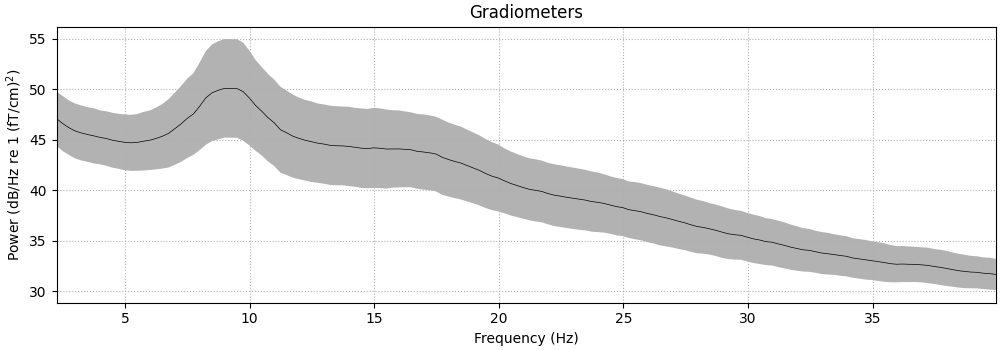```    Using multitaper spectrum estimation with 7 DPSS windows
Averaging across epochs...
```

Now, let’s take a look at the spatial distributions of the PSD, averaged across epochs and frequency bands.

```epochs.compute_psd().plot_topomap(ch_type="grad", normalize=False, contours=0)
```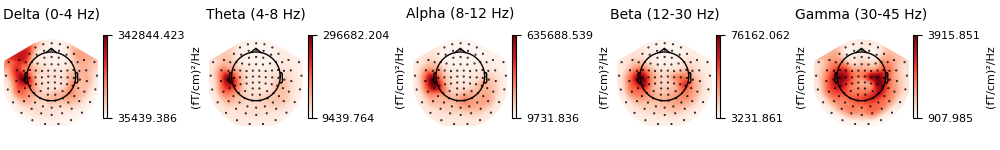```Using multitaper spectrum estimation with 7 DPSS windows
```

Alternatively, you can also create PSDs from `Epochs` methods directly.

Note

In contrast to the methods for visualization, the `compute_psd` methods do not scale the data from SI units to more “convenient” values. So when e.g. calculating the PSD of gradiometers via `compute_psd()`, you will get the power as `(T/m)²/Hz` (instead of `(fT/cm)²/Hz` via `plot_psd()`).

```_, ax = plt.subplots()
spectrum = epochs.compute_psd(fmin=2.0, fmax=40.0, tmax=3.0, n_jobs=None)
# average across epochs first
mean_spectrum = spectrum.average()
psds, freqs = mean_spectrum.get_data(return_freqs=True)
# then convert to dB and take mean & standard deviation across channels
psds = 10 * np.log10(psds)
psds_mean = psds.mean(axis=0)
psds_std = psds.std(axis=0)

ax.plot(freqs, psds_mean, color="k")
ax.fill_between(
freqs,
psds_mean - psds_std,
psds_mean + psds_std,
color="k",
alpha=0.5,
edgecolor="none",
)
ax.set(
xlabel="Frequency (Hz)",
ylabel="Power Spectral Density (dB)",
)
```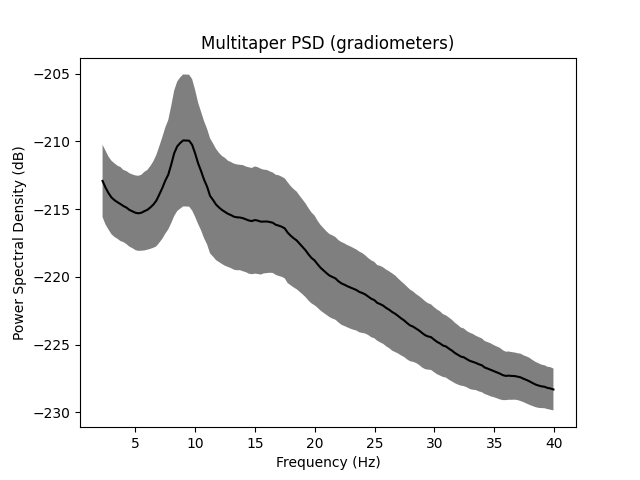```Using multitaper spectrum estimation with 7 DPSS windows
```

Notably, `mne.Epochs.compute_psd()` supports the keyword argument `average`, which specifies how to estimate the PSD based on the individual windowed segments. The default is `average='mean'`, which simply calculates the arithmetic mean across segments. Specifying `average='median'`, in contrast, returns the PSD based on the median of the segments (corrected for bias relative to the mean), which is a more robust measure.

```# Estimate PSDs based on "mean" and "median" averaging for comparison.
kwargs = dict(fmin=2, fmax=40, n_jobs=None)
psds_welch_mean, freqs_mean = epochs.compute_psd(
"welch", average="mean", **kwargs
).get_data(return_freqs=True)
psds_welch_median, freqs_median = epochs.compute_psd(
"welch", average="median", **kwargs
).get_data(return_freqs=True)

# Convert power to dB scale.
psds_welch_mean = 10 * np.log10(psds_welch_mean)
psds_welch_median = 10 * np.log10(psds_welch_median)

# We will only plot the PSD for a single sensor in the first epoch.
ch_name = "MEG 0122"
ch_idx = epochs.info["ch_names"].index(ch_name)
epo_idx = 0

_, ax = plt.subplots()
ax.plot(
freqs_mean,
psds_welch_mean[epo_idx, ch_idx, :],
color="k",
ls="-",
label="mean of segments",
)
ax.plot(
freqs_median,
psds_welch_median[epo_idx, ch_idx, :],
color="k",
ls="--",
label="median of segments",
)

ax.set(
title=f"Welch PSD ({ch_name}, Epoch {epo_idx})",
xlabel="Frequency (Hz)",
ylabel="Power Spectral Density (dB)",
)
ax.legend(loc="upper right")
```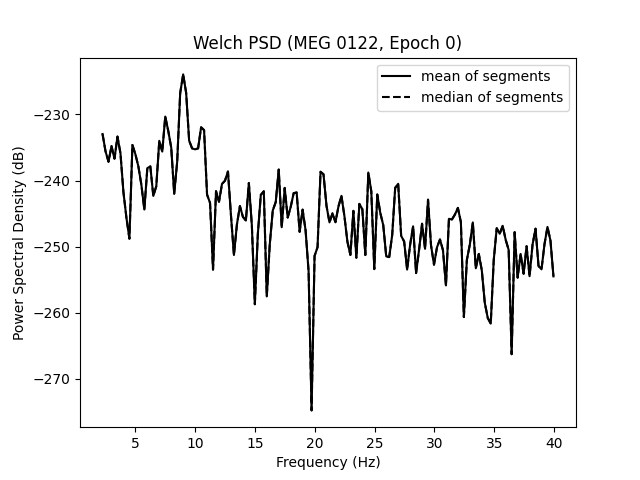```Effective window size : 4.005 (s)
Effective window size : 4.005 (s)
```

Lastly, we can also retrieve the unaggregated segments by passing `average=None` to `mne.Epochs.compute_psd()`. The dimensions of the returned array are `(n_epochs, n_sensors, n_freqs, n_segments)`.

```welch_unagg = epochs.compute_psd("welch", average=None, **kwargs)
print(welch_unagg.shape)
```
```Effective window size : 4.005 (s)
(28, 204, 152, 1)
```

## Time-frequency analysis: power and inter-trial coherence#

We now compute time-frequency representations (TFRs) from our Epochs. We’ll look at power and inter-trial coherence (ITC).

To this we’ll use the function `mne.time_frequency.tfr_morlet()` but you can also use `mne.time_frequency.tfr_multitaper()` or `mne.time_frequency.tfr_stockwell()`.

Note

The `decim` parameter reduces the sampling rate of the time-frequency decomposition by the defined factor. This is usually done to reduce memory usage. For more information refer to the documentation of `mne.time_frequency.tfr_morlet()`.

define frequencies of interest (log-spaced)

```freqs = np.logspace(*np.log10([6, 35]), num=8)
n_cycles = freqs / 2.0  # different number of cycle per frequency
power, itc = tfr_morlet(
epochs,
freqs=freqs,
n_cycles=n_cycles,
use_fft=True,
return_itc=True,
decim=3,
n_jobs=None,
)
```
```[Parallel(n_jobs=1)]: Done  17 tasks      | elapsed:    0.3s
[Parallel(n_jobs=1)]: Done  71 tasks      | elapsed:    1.1s
[Parallel(n_jobs=1)]: Done 161 tasks      | elapsed:    2.4s
```

## Inspect power#

Note

The generated figures are interactive. In the topo you can click on an image to visualize the data for one sensor. You can also select a portion in the time-frequency plane to obtain a topomap for a certain time-frequency region.

```power.plot_topo(baseline=(-0.5, 0), mode="logratio", title="Average power")
power.plot(, baseline=(-0.5, 0), mode="logratio", title=power.ch_names)

fig, axes = plt.subplots(1, 2, figsize=(7, 4), layout="constrained")
topomap_kw = dict(
ch_type="grad", tmin=0.5, tmax=1.5, baseline=(-0.5, 0), mode="logratio", show=False
)
plot_dict = dict(Alpha=dict(fmin=8, fmax=12), Beta=dict(fmin=13, fmax=25))
for ax, (title, fmin_fmax) in zip(axes, plot_dict.items()):
power.plot_topomap(**fmin_fmax, axes=ax, **topomap_kw)
ax.set_title(title)
```
•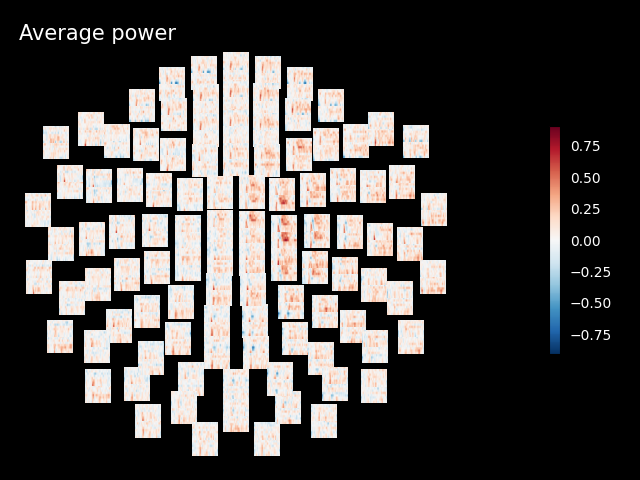•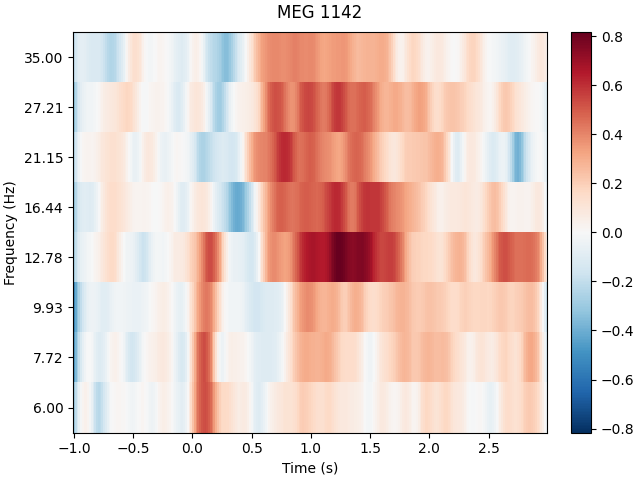•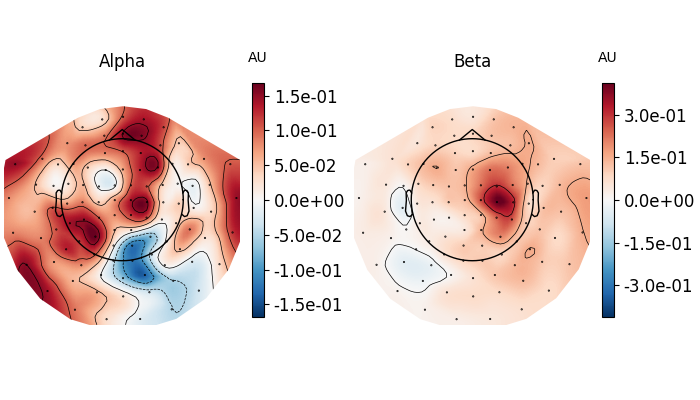```Applying baseline correction (mode: logratio)
Applying baseline correction (mode: logratio)
Applying baseline correction (mode: logratio)
Applying baseline correction (mode: logratio)
```

## Joint Plot#

You can also create a joint plot showing both the aggregated TFR across channels and topomaps at specific times and frequencies to obtain a quick overview regarding oscillatory effects across time and space.

```power.plot_joint(
baseline=(-0.5, 0), mode="mean", tmin=-0.5, tmax=2, timefreqs=[(0.5, 10), (1.3, 8)]
)
```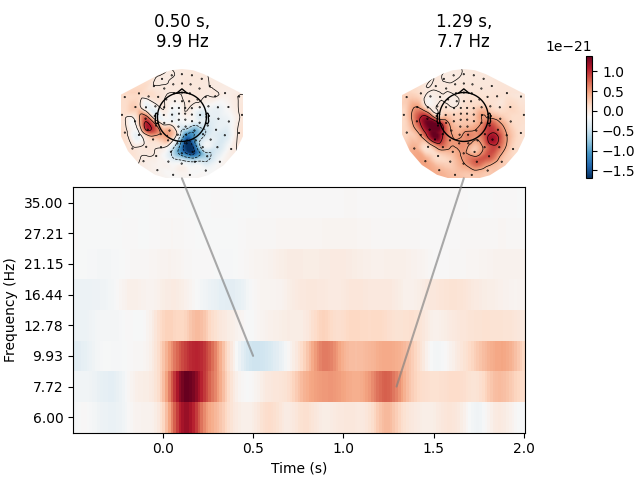```Applying baseline correction (mode: mean)
Applying baseline correction (mode: mean)
Applying baseline correction (mode: mean)
```

## Inspect ITC#

```itc.plot_topo(title="Inter-Trial coherence", vmin=0.0, vmax=1.0, cmap="Reds")
```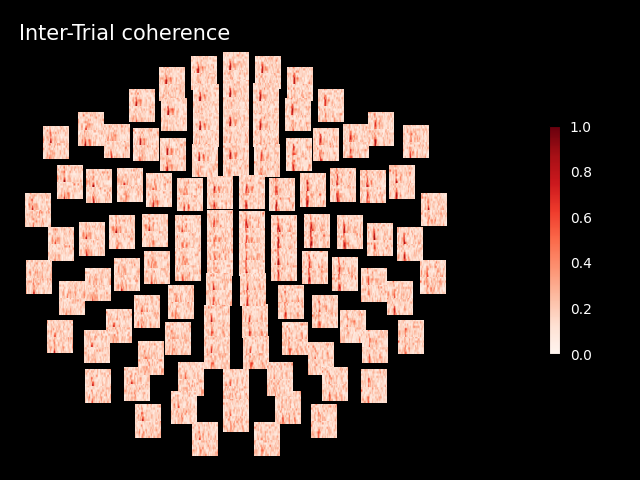```No baseline correction applied
```

Note

Baseline correction can be applied to power or done in plots. To illustrate the baseline correction in plots, the next line is commented:

```# power.apply_baseline(baseline=(-0.5, 0), mode='logratio')
```

## Exercise#

• Visualize the inter-trial coherence values as topomaps as done with power.

Total running time of the script: (0 minutes 39.345 seconds)

Estimated memory usage: 125 MB

Gallery generated by Sphinx-Gallery